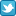Search DSS

Finding Data Analyzing Data Citing data

DSS lab consultation schedule
(Monday-Friday)
 Sep 1-Oct 16 By Zoom appt. here Oct 19-Dec 4 Virtual Zoom Walk-ins Dec 7-Jan 31 By Zoom appt. here Feb 1-April 30 Virtual Zoom Walk-ins May 3-Aug 31 By appt. here
For quick questions email data@princeton.edu.
*No appts. necessary during walk-in hrs.
Note: the DSS lab is open as long as Firestone is open, no appointments necessary to use the lab computers for your own analysis.HomeOnline HelpStatistical PackagesStata

# STATA

Stata is an interactive data analysis program which runs on a variety of platforms. Stata is installed on the Windows machines and Macs in OIT's public clusters and on the Windows machines in the DSS Data Lab.

## Introduction/data manipulation

• How can I get my data into Stata?
1. Using Stat/Transfer. Most common transfers are from SPSS/SAS to Stata.
2. From ASCII data. Use this link for resources when data is not in any propietary format (fixed-record form). A codebook or data layout is needed.
3. From Excel. Stata 12+ can read Excel files directly. In Stata go to File->Import->Excel (make sure to check 'import first row as variable names').
4. From Excel. Stata 11 or older you can either copy-and-paste. Or save the excel file as csv and import it using 'insheet'.
• Getting Started in Data Analysis. Stata tutorial to get started in data analysis (log file, set memory, describe and summarize data, frequencies, crosstabulations, descriptive statistics, scatterplots, histograms, recoding, renaming and creating new variables, merge, append and more), converting data from SPSS/SAS/Excel to Stata.
• Reshape. Reshaping data using an example from World Development Indicators (a commonly used dataset for macro level data)
• Merge/append data
• Running Stata on Unix. Running Unix Stata in text mode, Stata for Unix with an X-Windows interface, running large jobs in the background

## Statistical Analysis

• Linear regresssion. Tutorial on interpreting the outcome of linear regression, interactions and diagnostics: heteroskedasticity, functional form, predicted values, omitted-variable test, multicollinearity, outliers, normality, coefficients table (estto/esttab). Include how to present the regression output using outreg2 (in Word and Excel)
• Interpreting Stata Regression Output. Review of regression, R squared, significance.
• Using Dummy variables in a regression model
• Creating dummy variables.
• Event studies with Stata. Cleaning the data, calculating the event window, estimating normal performance, calculating the abnormal and cumulative abnormal returns, testins for significance.
• Descriptive statistics. Descriptive statistics using Stata, Excel and R.
• Fixed/random effects (panel data). Stata tutorial on panel data analysis showing fixed effects, random effects, hausman tests, test for time fixed effects, Breusch-Pagan Lagrange multiplier, contemporaneous correlation, cross-sectional dependence, testing for heteroskedasticity, serial correlation, unit roots
• Time series. Tutorial on setting the data as time series, use of lag operators, subsetting, interpreting correlograms, unit roots, cointegration, QLR or sup-Wald test, Granger causality, Chow test, test for serial correlation
• Logit/ordered logit regression. Tutorial on interpreting logit regression output and ordered logit regression output, odds ratios and estimation of probabilities.
• Factor analysis. Tutorial on factor analysis, predicting and interpreting output
• Multilevel analysis. Tutorial on multilevel analysis: varying intercept, varying coefficient model, varying slope model and postestimation
• Marginal effects, predicted probabilities. Predicted probabilities and marginal effects after (ordered) logit/probit using margins in Stata
• Differences-in-differences. A basic approach to d-i-d method
• Making nice output tables. Tutorial on using --outreg2-- to report regression output, descriptive statistics, frequencies and basic crosstabulations
• From NLS investigator to Stata